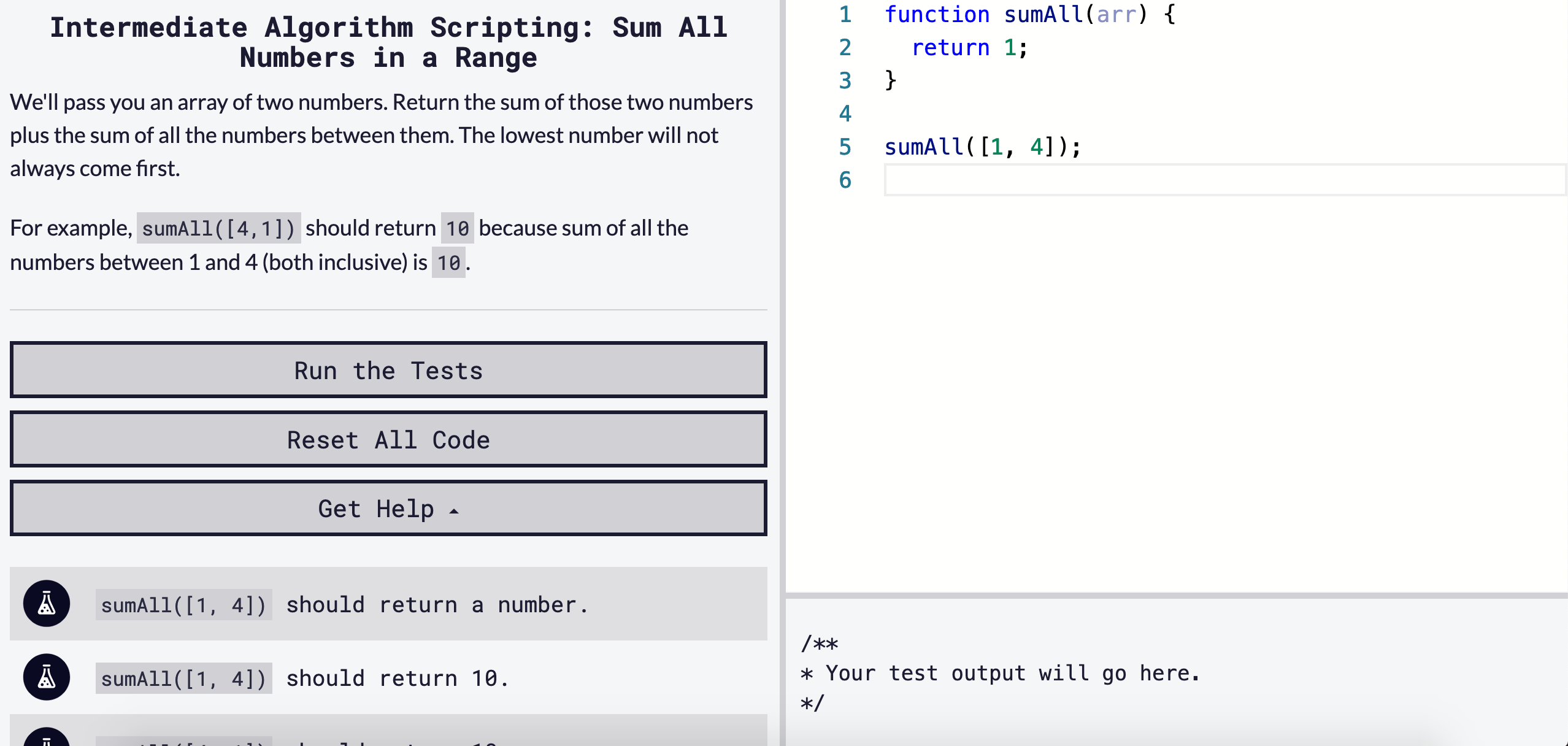## DEV Community

Viren B

Posted on • Originally published at virenb.cc

# Solving "Sum All Numbers in a Range" / freeCodeCamp Algorithm ChallengesLet's solve freeCodeCamp's intermediate algorithm scripting challenge, 'Sum All Numbers in a Range'.

### Starter Code

``````function sumAll(arr) {
return 1;
}

sumAll([1, 4]);
``````

### Instructions

We'll pass you an array of two numbers. Return the sum of those two numbers plus the sum of all the numbers between them. The lowest number will not always come first.

For example, `sumAll([4,1])` should return `10` because sum of all the numbers between 1 and 4 (both inclusive) is `10`.

### Tests

``````sumAll([1, 4]) should return a number.
sumAll([1, 4]) should return 10.
sumAll([4, 1]) should return 10.
sumAll([5, 10]) should return 45.
sumAll([10, 5]) should return 45.
``````

# Our Approach

Read everything first. Read the instructions clearly, read the starter code we're given, and read the tests and understand what has to be returned.

• The function takes one arguments, `arr` being an array.
• We need to sum the numbers plus all the numbers between the two.
• We need to return a number.

Now that we understand what we are given and what we want to output, let's see how we can solve this.

If you've completed the 'Functional Programming' section of freeCodeCamp, you would have been to some new array methods, `reduce()`, `filter()`, `map()`, and `sort()`.

Since we have to figure out the numbers in between the two given to us in `arr`, I would start by running `sort()` on `arr`, so `arr` would be the smallest number.

MDN Documentation: sort()

`arr.sort((a,b) => a - b);`

I will also declare a new empty array to add all the numbers in between into.

`let fullArr = [];`

In order to get all the numbers in between, I will create a for loop, and push each value into our `fullArr`.

`````` for (let i = arr; i <= arr; i++) {
fullArr.push(i);
}
``````

If we `sort()` as above then run our for loop, `fullArr` on `sumAll([1,4])` should be `[1, 2, 3, 4]`.

We now have all the numbers we need in an array. We just have to figure out how to add them all up.

Enter another new-er array method, `reduce()`.

'The reduce() method executes a reducer function (that you provide) on each element of the array, resulting in single output value.'

That sounds like what we want, a single output value.

MDN Documentation: reduce()

How to use reduce?

``````const array1 = [1, 2, 3, 4];

const reducer = (accumulator, currentValue) => accumulator + currentValue;

// 1 + 2 + 3 + 4
console.log(array1.reduce(reducer));
// expected output: 10
``````

Ensure you are returning a value!

So putting it all together with some pseudocode -

``````function sumAll(arr) {
create new empty array, fullArr
run sort method on array to arrange [small, big]
for loop on sorted arr
push each value into fullArr

fullArr, run reduce method, creating one single output integer value
return value
}
``````

# Our Solution

``````function sumAll(arr) {
let fullArr = [];
arr.sort((a,b) => a - b);
for (let i = arr; i <= arr; i++) {
fullArr.push(i);
}
let sum = fullArr.reduce((acc, currVal) => {return acc + currVal}, 0);
return sum;
}
``````

'Sum All Numbers in a Range' Challenge on fCC

freeCodeCamp

Donate to FCC!

Solution on my GitHubHi Viren,

Thanks for sharing! Just want to ask why not just add numbers in for loop. Like below

``````  let sum = 0;
for (let i = arr; i <= arr; i++) {
sum+= i
}
return sum
``````

Is there any problem with this method? Thanks.Nam Hoang Le

No. There is no problem in your methodology. But I think you assumed that `arr <= arr` ? Have your read description carefully?On your solution, you may change the original array destructively.

``````const arr = [5, 0]
sumAll(arr)  // 15
arr // [ 0, 5 ]
``````

To avoid this, you can use [...arr] or concat() method.

``````function sumAll(arr) {
arr = [...arr]
let fullArr = [];
arr.sort((a,b) => a - b);
for (let i = arr; i <= arr; i++) {
fullArr.push(i);
}
let sum = fullArr.reduce((acc, currVal) => {return acc + currVal}, 0);
return sum;
}

const arr = [5, 0]
sumAll(arr)  // 15
arr // [ 5, 0 ]
``````

And, my trials here:

``````const sumAll = ([a, b]) =>
closedRange(a, b)
.reduce(
(acc, e) => acc + e
, 0
)

const closedRange = (a, b) =>
a > b ? [...Array(a - b + 1).keys()]
.map(e => a - e )
: [...Array(b - a + 1).keys()]
.map( e => a + e )
``````

Or, more simply:

``````const sumAll = ([a, b]) =>
(a + b) * (Math.abs(a - b) + 1) / 2
``````